# Excel IFERROR function with formula examples

The tutorial shows how to use IFERROR in Excel to catch errors and replace them with a blank cell, another value or a custom message. You will learn how to use the IFERROR function with Vlookup and Index Match, and how it compares to IF ISERROR and IFNA.

"Give me the place to stand, and I shall move the earth," Archimedes once said. "Give me a formula, and I shall make it return an error," an Excel user would say. In this tutorial, we won't be looking at how to return errors in Excel, we'd rather learn how to prevent them in order to keep your worksheets clean and your formulas transparent.

## Excel IFERROR function - syntax and basic uses

The IFERROR function in Excel is designed to trap and manage errors in formulas and calculations. More specifically, IFERROR checks a formula, and if it evaluates to an error, returns another value you specify; otherwise, returns the result of the formula.

The syntax of the Excel IFERROR function is as follows:

IFERROR(value, value_if_error)

Where:

• Value (required) - what to check for errors. It can be a formula, expression, value, or cell reference.
• Value_if_error (required) - what to return if an error is found. It can be an empty string (blank cell), text message, numeric value, another formula or calculation.

For example, when dividing two columns of numbers, you may get a bunch of different errors if one of the columns contains empty cells, zeros or text.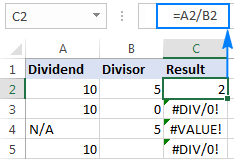To prevent that from happening, use the IFERROR function to catch and handle errors the way you want.

#### If error, then blank

Supply an empty string (") to the value_if_error argument to return a blank cell if an error is found:

`=IFERROR(A2/B2, "")`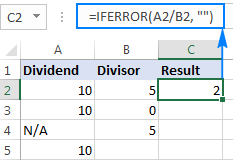#### If error, then show a message

You can also display your own message instead of Excel's standard error notation:

`=IFERROR(A2/B2, "Error in calculation")`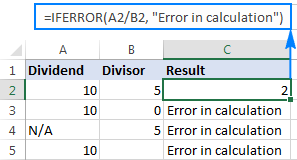### 5 things you should know about Excel IFERROR function

1. The IFERROR function in Excel handles all error types including #DIV/0!, #N/A, #NAME?, #NULL!, #NUM!, #REF!, and #VALUE!.
2. Depending on the contents of the value_if_error argument, IFERROR can replace errors with your custom text message, number, date or logical value, the result of another formula, or an empty string (blank cell).
3. If the value argument is a blank cell, it is treated as an empty string (''') but not an error.
4. IFERROR was introduced in Excel 2007 and is available in all subsequent versions of Excel 2010, Excel 2013, Excel 2016, Excel 2019, Excel 2021, and Excel 365.
5. To trap errors in Excel 2003 and earlier versions, use the ISERROR function in combination with IF, as shown in this example.

## IFERROR formula examples

The following examples show how to use IFERROR in Excel in combination with other functions to accomplish more complex tasks.

### Excel IFERROR with Vlookup

One of the most common uses of the IFERROR function is telling the users that the value they are searching for does not exist in the data set. For this, you wrap a VLOOKUP formula in IFERROR like this:

If the lookup value is not in the table you are looking in, a regular Vlookup formula would return the #N/A error: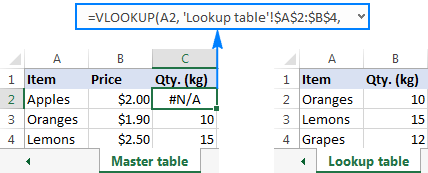For your users' piece of mind, wrap VLOOKUP in IFERROR and display a more informative and user-friendly message:

`=IFERROR(VLOOKUP(A2, 'Lookup table'!\$A\$2:\$B\$4, 2,FALSE), "Not found")`

The screenshot below shows this Iferror formula in Excel: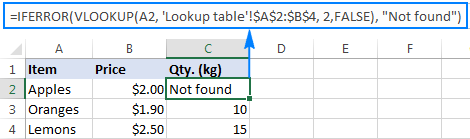If you'd like to trap only #N/A errors but not all errors, use the IFNA function instead of IFERROR.

For more Excel IFERROR VLOOKUP formula examples, please check out these tutorials:

### Nested IFERROR functions to do sequential Vlookups in Excel

In situations when you need to perform multiple Vlookups based on whether the previous Vlookup succeeded or failed, you can nest two or more IFERROR functions one into another.

Supposing you have a number of sales reports from regional branches of your company, and you want to get an amount for a certain order ID. With A2 as the lookup value in the current sheet, and A2:B5 as the lookup range in 3 lookup sheets (Report 1, Report 2 and Report 3), the formula goes as follows:

`=IFERROR(VLOOKUP(A2,'Report 1'!A2:B5,2,0),IFERROR(VLOOKUP(A2,'Report 2'!A2:B5,2,0),IFERROR(VLOOKUP(A2,'Report 3'!A2:B5,2,0),"not found")))`

The result will look something similar to this: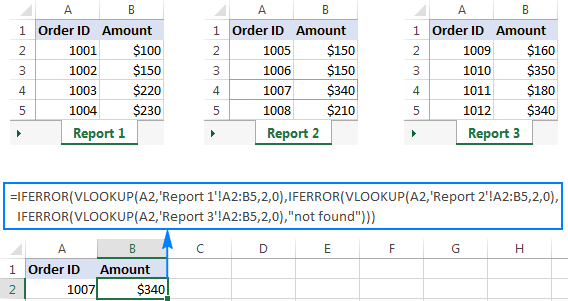For the detailed explanation of the formula's logic, please see How to do sequential Vlookups in Excel.

### IFERROR in array formulas

As you probably know, array formulas in Excel are meant to perform multiple calculations within a single formula. If you supply an array formula or expression that results in an array in the value argument of the IFERROR function, it'd return an array of values for each cell in the specified range. The below example shows the details.

Let's say, you have Total in column B and Price in column C, and you want to calculate Total Quantity. This can be done by using the following array formula, which divides each cell in the range B2:B4 by the corresponding cell of the range C2:C4, and then adds up the results:

`=SUM(\$B\$2:\$B\$4/\$C\$2:\$C\$4)`

The formula works fine as long as the divisor range does not have zeros or empty cells. If there is at least one 0 value or blank cell, the #DIV/0! error is returned: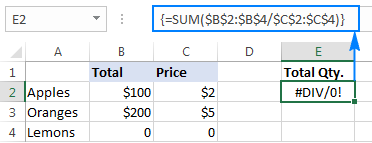To fix that error, simply do the division within the IFERROR function:

`=SUM(IFERROR(\$B\$2:\$B\$4/\$C\$2:\$C\$4,0))`

What the formula does is to divide a value in column B by a value in column C in each row (100/2, 200/5 and 0/0) and return the array of results {50; 40; #DIV/0!}. The IFERROR function catches all #DIV/0! errors and replaces them with zeros. And then, the SUM function adds up the values in the resulting array {50; 40; 0} and outputs the final result (50+40=90).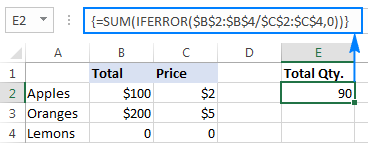Note. Please remember that array formulas should be completed by pressing the Ctrl + Shift + Enter shortcut.

## IFERROR vs. IF ISERROR

Now that you know how easy it is to use the IFERROR function in Excel, you may wonder why some people still lean towards using the IF ISERROR combination. Does it have any advantages compared to IFERROR? None. In the bad old days of Excel 2003 and lower when IFERROR did not exist, IF ISERROR was the only possible way to trap errors. In Excel 2007 and later, it's just a bit more complex way to achieve the same result.

For instance, to catch Vlookup errors, you can use either of the below formulas.

In Excel 2007 - Excel 2016:

In all Excel versions:

Notice that in the IF ISERROR VLOOKUP formula, you have to Vlookup twice. In plain English, the formula can be read as follows: If Vlookup results in error, return "Not found", otherwise output the Vlookup result.

And here is a real-life example of an Excel If Iserror Vlookup formula:

`=IF(ISERROR(VLOOKUP(D2, A2:B5,2,FALSE)),"Not found", VLOOKUP(D2, A2:B5,2,FALSE ))`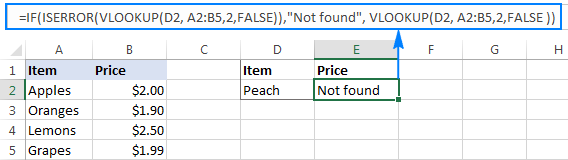## IFERROR vs. IFNA

Introduced with Excel 2013, IFNA is one more function to check a formula for errors. Its syntax is similar to that of IFERROR:

IFNA(value, value_if_na)

In what way is IFNA different from IFERROR? The IFNA function catches only #N/A errors while IFERROR handles all error types.

In which situations you may want to use IFNA? When it is unwise to disguise all errors. For example, when working with important or sensitive data, you may want to be alerted about possible faults in your data set, and standard Excel error messages with the "#" symbol could be vivid visual indicators.

Let's see how you can make a formula that displays the "Not found" message instead of the N/A error, which appears when the lookup value is not present in the data set, but brings other Excel errors to your attention.

Supposing you want to pull Qty. from the lookup table to the summary table as shown in the screenshot below. Using the Excel Iferror Vlookup formula would produce an aesthetically pleasing result, which is technically incorrect because Lemons do exist in the lookup table: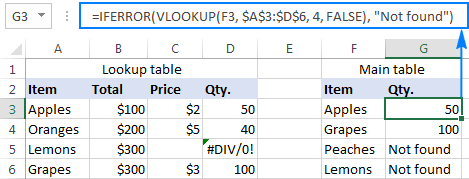To catch #N/A but display the #DIV/0 error, use the IFNA function in Excel 2013 and Excel 2016:

`=IFNA(VLOOKUP(F3,\$A\$3:\$D\$6,4,FALSE), "Not found")`

Or, the IF ISNA combination in Excel 2010 and earlier versions:

`=IF(ISNA(VLOOKUP(F3,\$A\$3:\$D\$6,4,FALSE)),"Not found", VLOOKUP(F3,\$A\$3:\$D\$6,4,FALSE))`

The syntax of the IFNA VLOOKUP and IF ISNA VLOOKUP formulas are similar to that of IFERROR VLOOKUP and IF ISERROR VLOOKUP discussed earlier.

As shown in the screenshot below, the Ifna Vlookup formula returns "Not found" only for the item that is not present in the lookup table (Peaches). For Lemons, it shows #DIV/0! indicating that our lookup table contains a divide by zero error: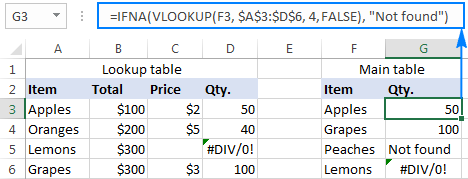## Best practices for using IFERROR in Excel

By now you already know that the IFERROR function is the easiest way to catch errors in Excel and mask them with blank cells, zero values, or custom messages of your own. However, that does not mean you should wrap each and every formula with error handling. The following simple recommendations may help you keep the balance.

1. Don't trap errors without a reason.
2. Wrap the smallest possible part of a formula in IFERROR.
3. To handle only specific errors, use an error handling function with a smaller scope:
• IFNA or IF ISNA to catch only #N/A errors.
• ISERR to catch all errors except for #N/A.

This is how you use the IFERROR function in Excel to trap and handle errors. To have a closer look at the formulas discussed in this tutorial, you are welcome to download our sample IFERROR Excel workbook. I thank you for reading and hope to see you on our blog next week.

## You may also be interested in

### 47 comments to "Excel IFERROR function with formula examples"

1.Rose says:

Hello, I need help with these formula, I need to create a word, 1 lower first letter from B3, 1 lower first letter from C3, and 4 numbers from right of A3. I used this formula, but it does not work. Thank you!

=IF(ISERROR(FIND(" ",B3)),LOWER(LEFT,1)),CONCATENATE(LOWER(LEFT(B3,1)),(FIND(" ",B3)+1),1)),IF(ISERROR(FIND(" ",C3)),LOWER(LEFT(C3,1)),CONCATENATE(LOWER(LEFT(C3,1)),(FIND(" ",C3)+1),1)),IF(ISERROR(FIND(" ",A3)),LOWER(RIGHT(A3,4)),CONCATENATE(LOWER(RIGHT(3,4)),(FIND(" ",A3)+1),1))

2.wendy says:

Hello, i have a worksheet which has a formula referencing to calculating a discounted price, =F9*(1-H9). I have rows that do not have any price or % present resulting in my calucation giving me the #VALUE! error when i drag the formula down. is there any way i can use the IFERROR? i have tried a few different ways but i cannot get it to work. I do not want to hide as there are totals at the end of the rows which also give the same error. thanks for any help

3.Shidhesh Naik says:

Very beautifully explained. I have got a complete grip on the formula now. Thanks for sharing the information and keep up the good work.

4.Svetlozar Todorov says:

Hi there,
It is great you can lean on real pros in the field.
Trying to figure out solution for the following problem.
1. Replacing the consecutives appearances of #N/A value in a column with dynamically incremental values for each appearance of #N/A down the column starting with particular number.
For example:
1st #N/A appearance to be replaced with 10000
2nd #N/A appearance to be replaced with 10001 and so on.

To a moment all the #N/A appearances in the column of the data are replaced with those consecutive numbers.

I know I am close, but I am missing something.

Svetlozar Todorov

•Alexander Trifuntov (Ablebits Team) says:

Hello!
Maybe this formula will work for you.

=IFNA(VLOOKUP(F2,\$A\$3:\$B\$13,2,FALSE), COUNTIF(\$D\$2:D2,">9999")+10000)

It is assumed that it is written in cell D3

=IFNA(VLOOKUP(F2,\$A\$3:\$B\$13,2,FALSE),COUNTIF(\$D\$2:D2,">9999")+10000)

•Svetlozar Todorov says:

Hi Alexander,
Thank you for your advice on the formula that may work out the problem.
I have tried to adjust for the case I have, but did not get the intended result.
Let me widen the, details as those I have stated are probably not sufficient to have the whole picture clear.
So we have a result column that compares 2 datasheets by let say attribute Code ID:
The first database has IDs for the items that are assigned in incremental manner: 1,2,3 and so on. All of the items there has Code IDs assigned.
The second Database consist of items that are mixed with items that are part of the first data base and have Code ID already assigned and new items that are freshly composed and needs to have New ID's assigned. In the second data base are not presented all of the items in the first database.
The ultimate goal is to assign new Code IDs for the items in the Second database that are newly composed with starting point "x+1" where "x" is the last Code ID in the first database.

This can be worked with filters I presume, but the elegance of getting the results with incremental values by formula is more appealing as solution.

Thank you in advance for the efforts to present something new with the routine of power knowledge you and the team have.
After I have tried to adjust the formula I ended up with warning saying: " There are one or more circular reference where formula refers to itself directly or indirectly and may calculate improperly".
I ended up with all the #N/A found in the database replaced with the first value of the first row that was compared from the first database.
I have tried 2 ways, but did returned result for all of the empty cells as value 13080.
Example of the formula:
1st attempt
=IFNA(VLOOKUP(H2,'[Export Goods Microinvest 15_02_22.xlsx]Microinvest'!\$A:\$A,1,FALSE),COUNTIF('[Export Goods Microinvest 15_02_22.xlsx]Microinvest'!\$A:\$A,">13079")+13080)
2nd attempt
=IFNA(VLOOKUP(H3,'[Export Goods Microinvest 15_02_22.xlsx]Microinvest'!\$A:\$A,1,FALSE),COUNTIF(H:H,">13079")+13080)
Column H holds the Code IDs and Empty cells (This are the second database lines)
Column A is holding Code IDs of the first database.
13079 is the last CodeID in the first database.
I presume I am not using COUNTIF correctly but run out of ideas to get this sorted.

Svetlozar Todorov

•Alexander Trifuntov (Ablebits Team) says:

Hello!
If the first table is A3:A13 and the second table is D3:D13 then try the formula in cell E3

=IFERROR(VLOOKUP(D3,\$A\$3:\$B\$13,2,FALSE),MAX(MAX(\$B\$3:\$B\$13)+1,MAX(\$E\$2:E2)+1))

Сopy it down along the column

•Svetlozar Todorov says:

Hi Alexander,

I have edited the formula, while I was trying to figure your logic out.

What is the point of a range A:B? I have edited to A:A,
Same for the second part :
MAX(MAX(\$B\$3:\$B\$13) - That was edited to A:A and the next part
"MAX(\$E\$2:E2)+1))" - is this correct the formula is column E. What is this part serving, as the formula is supposed to be filled in this column.
The result of different edits is not populating incremental values for the missing CodeID, but is pasting the same value which is the highest in the column + 1.
Appreciate the efforts!

Thanks!

Svetlozar Todorov

•bHAGATH says:

I need formula, to extract only 3 columns to separate sheet

Considering Product type located in Q column, it has to get Column D Assest ID & Price which in T column into new sheet.

Everything to be extract with formula only from Main sheet

•Alexander Trifuntov (Ablebits Team) says:

Hi!
I can't see your data so I can't understand what you want to do. Explain in more detail.

•Bhagath says:

I have a bulk data, out of that i need to pull only Column D, Q, T

We need to consider Column Q as Main and have to extract D & T

Column Q is Product type
Column D Asset ID
Column T Price

•Alexander Trifuntov (Ablebits Team) says:

Hi!
To solve your problem, I would recommend using the FILTER function. You can get a range of data based on the criteria that you specify. However, no Excel function can retrieve data from non-adjacent ranges.

5.Stacey says:

need some help with my formula.

Once the cell returns the max number indicated in my vlookup statement (the 13 in the statement) i want to have all remaining cells be blank. how do i do that? the "" isn't doing that like i thought it would. it just keeps showing the max (i.e max is 13.96 and all remaining cells after this continue to show 13.96)

=IFERROR(IF(L4*1.02>((VLOOKUP(\$A4,yoe,13,FALSE))),VLOOKUP(\$A4,yoe,13,FALSE),L4*1.02),"")

•Alexander Trifuntov (Ablebits Team) says:

Hi!
It is very difficult to check a formula that contains unique references to your workbook worksheets. It is also not clear what means "all remaining cells". Describe your task in more detail.

6.Saravanan Ins says:

(Table 1)
Start Page | End Page
1 3
4 5
6 26
27 27
28 28
29 29
30 32

(Table 2)
Start Page | End Page
1 3
4 5
6 6
7 26
27 27
28 29
29 29
30 32

How to Compare Two table use formula?
I expect result for below

Table 1 Table 2
1 3 1 3
4 5 4 5
6 7 6 26
8 26 Error
27 27 27 27
28 28 28 29 Error
29 29 29 29
30 32 30 32

7.SANDEEP MORESHWAR KELKAR says:

=IF(ISNUMBER(SEARCH("Drumstick PKM 1 50 gm",Q2)),2,IF(ISNUMBER(SEARCH("Bajra No",Q2)),3,IF(ISNUMBER(SEARCH("induce",Q2)),4,IF(ISNUMBER(SEARCH("pusa",Q2)),8,IF(ISNUMBER(SEARCH("ajay",Q2)),9,IF(ISNUMBER(SEARCH("Humic Acid Roota 1 kg",Q2)),10,IF(ISNUMBER(SEARCH("Humic Acid Roota 500 gm",Q2)),11,IF(ISNUMBER(SEARCH("Cucumber",Q2)),12,IF(ISNUMBER(SEARCH("ARCH 930",Q2)),13,IF(ISNUMBER(SEARCH("latika",Q2)),14,IF(ISNUMBER(SEARCH("2338 250",Q2)),16,IF(ISNUMBER(SEARCH("lucky",Q2)),17,IF(ISNUMBER(SEARCH("bhim",Q2)),18,IF(ISNUMBER(SEARCH("karishma",Q2)),19,IF(ISNUMBER(SEARCH("BAIF",Q2)),21,IF(ISNUMBER(SEARCH("Arka",Q2)),22,IF(ISNUMBER(SEARCH("Alamdar 51",Q2)),23,IF(ISNUMBER(SEARCH("kajol",Q2)),25,IF(ISNUMBER(SEARCH("Bijankur",Q2)),28,IF(ISNUMBER(SEARCH("Auskelp 500 ml",Q2)),29,IF(ISNUMBER(SEARCH("Supplement Vimicon",Q2)),30,IF(ISNUMBER(SEARCH("Aspartical",Q2)),31,IF(ISNUMBER(SEARCH("Lucerne RL88",Q2)),33,IF(ISNUMBER(SEARCH("Okra 2338 100",Q2)),34,IF(ISNUMBER(SEARCH("Subabul",Q2)),35,IF(ISNUMBER(SEARCH("PKM 1 250",Q2)),36,IF(ISNUMBER(SEARCH("Shevari CMS 800",Q2)),37,IF(ISNUMBER(SEARCH("COFS 29 50",Q2)),38,IF(ISNUMBER(SEARCH("COFS 29 200",Q2)),39,IF(ISNUMBER(SEARCH("Agathi 400",Q2)),40,IF(ISNUMBER(SEARCH("Agathi 800",Q2)),41,IF(ISNUMBER(SEARCH("Shevari CMS 400",Q2)),42,0))))))))))))))))))))))))))))))))

how will i reduce this if formula

8.Mariyam Zaidi says:

IF(ISERROR(IF((E120/D120)>=2,0,IF(AND((E120/D120)1),(1-(E120/D120))+1,IF((E120/D120)<0,0,IF((E120/D120)=2,0,IF(AND((E120/D120)1),(1-(E120/D120))+1,IF((E120/D120)<0,0,IF((E120/D120)<=1,(E120/D120))))))

Can someone please explain to me why are we using this big formula for calculating forecast accuracy??

Simple formula could be IFERROR(E120/D120,0) where E120 is actual forecast lets say 1 and D120 is forecast lets say 1

9.custom box says:

This is really very helpful for bloggers
You’re doing a great job Man, Keep it up.

10.Chris says:

I am trying to do a simple add and average of a column that is in percentage format. For example:

Column
50%
100%
50%
80%

Average Score: 70%

I keep getting a DIV 0 error

•Alexander Trifuntov (Ablebits Team) says:

Hi!
Your error means division by zero. What formula are you using?

11.Muhammadh says:

=IFERROR(MATCH(E10,item_ID,0)+2,'''') The above formula is showing a error message in my Excel 2016. That formula is correct and it worked in another PC which having Excel 2013. Please help me to get rid of this error.

•Alexander Trifuntov (Ablebits Team) says:

Hello!
Your formula has a reference to the named range item_ID. I think your file does not have it. Create a named range item_ID in the desired cells.

12.brownchinito says:

=IFERROR((U7/\$B7)-1,0)

Question what is the purpose of -1?

13.Stephen Ochieng says:

Albeits.com is dong a great job
bravo!!

14.Jagjit Rai says:

=IFERROR(BK4-BM4,"N/A")&IF(BK4>BM4," Over HC")
Sorry I forgot to show you my Formula.
Regards,
Jagjit

15.Jagjit Rai says:

1 HC Max 36 2 Current HC 3 Performance-(THese are 3 headings)
17 15 2 Over HC Correct answer
Not played N/A N/A Over HC In correct- only need (N/A) Remove Over HC
36 36 0FALSE Incorrect- only need (0) Remove FALSE
Deae Sirs,
I have 3 columns as shown above ,and am subtracting column 2 from column 1 to get the following answers in column 3:-
1)Result to show "Nr Over HC" as 1 or 2 or 3 with remaks as " nr Over HC"-this is correct
2)Result to show N/A only But I get "N/A Over HC" together-This is not Correct.
3)Result to show 0 only But I get 0FALSE- This is not correct
I will be grateful if yu can help please.
Regards,
Jagjit

16.David says:

I have a formula that is working, but it is not giving me a true picture.
This is the current cell(B5) formula: =IFERROR(IF(AND(AA5="",AJ5=""),"",\$A\$2-\$AJ5),"")
I need this cell(B5) to be BLANK if the adjacent cell(C5) is populated. C5 has a formula in it that gives me a number or a BLANK.

•Alexander Trifuntov (Ablebits Team) says:

Hello David!
If I understand your task correctly, the following formula should work for you:

=IF(C5<>"",IFERROR(IF(AND(AA5="",AJ5=""),"",\$A\$2-\$AJ5),""),"")

I hope this will help

17.Munawar says:

A1 1
A2 2
A3 ERROR
A4 4
if we do sum(A1:A4), sum should'nt work, its there any way

•Alexander Trifuntov (Ablebits Team) says:

Hello Munawar!
The value in Cell A3 has been received as a result of the incorrect performance of some formula. It is impossible to use this cell with an error in Excel formulas.
To avoid such situations, it is necessary to use the IFERROR function.
You can also see examples of the IFERROR function usage there. Undoubtedly, they will be helpful to you.

18.saravana says:

Nice Information to grow the Knowledge.

19.Babu says:

Dear All,
Kindly help me for this below table looking for formula. Next sheet if i click the any names it should get the data...
Sl. No. NAMES DAY DATE Business Purchase Amount
1 A Monday 2-Sep-19 1000
2 B Tuesday 4-Sep-19 1050
3 C Wednesday6-Sep-19 1500
4 D Thursday8-Sep-19 950
5 E Friday 10-Sep-19 1360
6 A Saturday12-Sep-19 490
7 B Sunday 14-Sep-19 1485
8 C Monday 16-Sep-19 620
9 D Tuesday 18-Sep-19 9563
10 E Wednesday20-Sep-19 623

20.Ishtiaq Ali Syed says:

Please I have to know about ifferror() ,how to apply in excel 2009,?

21.Pet says:

Hello everyone - just need some help please
COLUMN A = Response Sent Date
COLUMN B = Last Update Date
OUTPUT - I need to calculate how many working days from COLUMN B; however if COLUMN B is Blank or no date, it will refer to COLUMN A's date

22.Josephine says:

What is the formula of; when u create another excel for overall charts and u need to sum or average it, and make sure that theres no result yet will not appear on the chart u create.

23.Shravan says:

Hello Sir/Mam,

i am create a scorecard for my employ's. i have fixed target and tracking daily basis achievement.
for example.

Target = 6, (E2) Column, Achievement = 0, (F2) Column. i am used if formula =IFERROR (IF((20*(E2/F2)>20),20,(20*(E2/F2))),"20.0")

value showing is (20.0) but i am use sum function Parameter-1 value is (20.0) and parameter-2 value is (15.0) both value sum = (35.0) but showing is (15.0)

24.Nabeel says:

25.Dilip Dighe says:

Dear Sir/Mam
very helpful & thankful yours guideline & thank u so much....

I Create one table... I M nt sure which is formula this table...
plz. help me..

Table (FOR EXAMPLE)

1 A B C D E F
2 Sr.No Name Item Qty. Rate Total
3 1 Abc Box 50 100 5000
4 2 Abc Box 20 50 1000
5 3 Cbd Box 20 50 1000
6 4 Dcd Box 20 50 1000

I Enter (Lookup Value)Other Sheet - Sr.No.1 &
Result is :- (TABLE)Below

Enter No. 1
Name = Abc (Vlookup Value)
1 A B C D
2 Item Qty. Rate Total
3 Box 50 100 5000
4 Box 20 50 1000

plz. help me..

Thnking you
Dilip

26.Shilpa says:

Nice information it helps all computer science students

27.Patrick says:

Great synopsys. Thanks

28.Manny says:

Hello. I have a file with data in 4 columns. Some of the data may be repeated between columns. How can I create a list in column 5 with unique numbers from the other 4?

Any help is greatly appreciated. Thank you!

29.Renny says:

Please Help. I need to track # of homes by Month (Column), by Community # (Row), from my boss's worksheet (2018 starts.xlsx) to my own. It pulls in the right information, but every time I open my spreadsheet, my computer can't seem to function properly. an Intern helped with the formula, and she's unreachable.

Any help is greatly appreciated. Thank you!

=IFERROR(IF(LEN(INDEX('[2018 starts.xlsx]Labor North'!\$1:\$1048576,MATCH(\$B7,'[2018 starts.xlsx]Labor North'!\$C:\$C,0),MATCH(P\$5,'[2018 starts.xlsx]Labor North'!\$2:\$2,0))),INDEX('[2018 starts.xlsx]Labor North'!\$1:\$1048576,MATCH(\$B7,'[2018 starts.xlsx]Labor North'!\$C:\$C,0),MATCH(P\$5,'[2018 starts.xlsx]Labor North'!\$2:\$2,0)),""),"")

30.Ananad Kumar says:

Dear Sir/Team
I have make an excel sheet of my business ledger. But in this worksheet "Iferror & Index" formula not working after 499 rows, and nothing shown any result after 499 rows. Is this formula only worked upto 499 rows or not? Can I increase the rows above 499? If I can increase the row after 499, then what should I do? Please tell me.
•Gennady Terekhov (Ablebits Team) says:
31.rohan says: